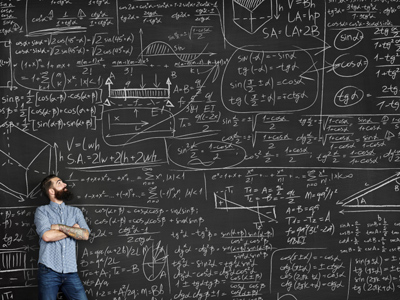There are three particularly challenging questions in this quiz.

# Number Sequences 7

This Math quiz is called 'Number Sequences 7' and it has been written by teachers to help you if you are studying the subject at elementary school. Playing educational quizzes is an enjoyable way to learn if you are in the 3rd, 4th or 5th grade - aged 8 to 11.

It costs only \$12.50 per month to play this quiz and over 3,500 others that help you with your school work. You can subscribe on the page at Join Us

Number sequences involve rules and finding them can be a bit of a challenge at times! This is our final elementary school Math quiz on number sequences. Some of these questions are particularly tricky, so take your time and think carefully before choosing your answer.

You may think that number sequences are not an important part of Maths. The thing with numbers is, the more you are familiar with how they work, the better you'll be in whichever career you choose. Plenty of careers require a solid knowledge of numbers, such as any of the sciences, and if you want a good career then learn your numbers!

Time for the quiz. You may find a pencil & paper handy for these questions. Good luck.

1.
Find the missing term.
-17, -11, -5, X, ...
1
0
-1
2
The next term is got from the previous term by adding 6, e.g. -17 + 6 = -11 and so on
2.
Find the missing term.
10, 100, 1,000, X, ...
10,000,000
10,000
100,000
1,000,000
The next term is got from the previous term by multiplying by 10, e.g. 10 × 10 = 100 and so on
3.
Find the missing term.
1, 1, 2, 3, 5, 8, X, ...
11
13
12
10
This is the famous Fibonacci sequence. Each term is formed by the sum of the previous TWO terms: 1 + 1 = 2, 1 + 2 = 3, 2 + 3 = 5, 3 + 5 = 8, 5 + 8 = 13 and so on
4.
Find the missing term.
0, 4, 18, 48, X, 180, ...
96
120
100
90
The rule for the nth term = n3 - n2. Put in the values of 1, 2, 3, 4, ... in turn and and see for yourself how the sequence is produced
5.
Find the missing term.
-4, 3, 10, X, ...
17
16
15
14
The next term is got from the previous term by adding 7, e.g. -4 + 7 = 3 and so on
6.
Find the missing term.
-10, -7, -4, X, ...
2
0
1
-1
The next term is got from the previous term by adding 3, e.g. -7 + 3 = -4 and so on
7.
Find the missing term.
39, 52, 65, X, ...
79
77
78
81
The next term is got from the previous term by adding 13, e.g. 39 + 13 = 52 and so on
8.
Find the missing term.
3, 7, 11, X, ...
14
16
15
17
The next term is got from the previous term by adding 4, e.g. 3 + 4 = 7 and so on
9.
Find the missing term.
1, 8, 27, X, ...
81
49
36
64
The terms of this sequence are the counting numbers cubed: 13 = 1; 23 = 8; 33 = 27; 43 = 64; 53 = 125 and so on
10.
Find the missing term.
79, 95, X, 127, ...
111
110
103
115
The next term is got from the previous term by adding 16, e.g. 79 + 16 = 95 and so on
Author:  Frank Evans